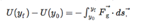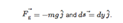# Problem: Consider a uniform gravitational field (a fair approximation near the surface of a planet). FindWhereExpress your answer in terms of m, g, y0, and y­f.

###### FREE Expert Solution

For a uniform gravitational field:

$\begin{array}{rcl}\mathbf{U}\mathbf{\left(}{\mathbf{y}}_{\mathbf{f}}\mathbf{\right)}\mathbf{-}\mathbf{U}\mathbf{\left(}{\mathbf{y}}_{\mathbf{0}}\mathbf{\right)}& \mathbf{=}& \mathbf{-}{\mathbf{\int }}_{{\mathbf{y}}_{\mathbf{0}}}^{{\mathbf{y}}_{\mathbf{f}}}{\mathbf{F}}_{\mathbf{g}}\mathbf{·}\mathbf{d}\mathbf{s}\end{array}$

But gravitational potential energy, U = mgh

92% (32 ratings)###### Problem Details

Consider a uniform gravitational field (a fair approximation near the surface of a planet). FindWhere